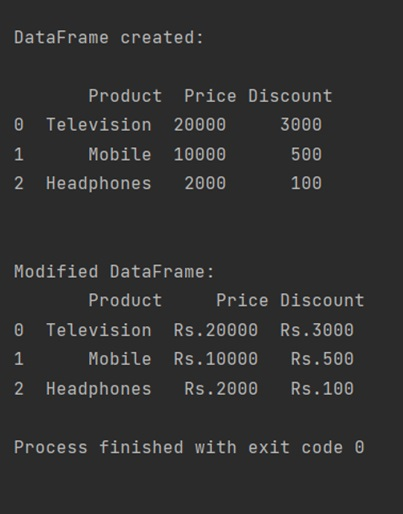# How to apply a function to two columns of Pandas DataFrame?

In this article, we are going to learn how to apply a function to more than one column in a DataFrame?
Submitted by Pranit Sharma, on April 25, 2022

In Pandas, we can achieve this task by using DataFrame.apply() method.

## Pandas.DataFrame.apply() Method

This function itself takes a function as a parameter which has to be applied on the columns. To apply the function to multiple columns, we just need to define the name of the columns inside pandas.DataFrame.apply() method.

Syntax:

```DataFrame.apply(parameters)
```

Parameter(s):

• It takes the function which has to be applied on the column values.
• It takes several other optional parameters like axis, raw, result_type, **kwds.

To work with Python Pandas, we need to import the pandas library. Below is the syntax,

```import pandas as pd
```

Let us understand with the help of an example.

```# Importing pandas package
import pandas as pd

# Defining a function for modification of
# column values
def function(value):
# Adding string ='Rs.' before the value
return 'Rs.'+ value

# Creating a list of dictionary
data = {
'Price':['20000','10000','2000'],'Discount':['3000','500','100']
}

# Converting a DataFrame
df=pd.DataFrame(data)

# Display the DataFrame
print("\nDataFrame created:\n\n",df,"\n\n")

# Using the apply() method to call the function: function
df[['Price','Discount']] = df[['Price','Discount']].apply(function)

# Display modified DataFrame
print("Modified DataFrame:\n",df)
```

Output:What's New (MCQs)

Top Interview Coding Problems/Challenges!

IncludeHelp's Blogs

Languages: » C » C++ » C++ STL » Java » Data Structure » C#.Net » Android » Kotlin » SQL
Web Technologies: » PHP » Python » JavaScript » CSS » Ajax » Node.js » Web programming/HTML
Solved programs: » C » C++ » DS » Java » C#
Aptitude que. & ans.: » C » C++ » Java » DBMS
Interview que. & ans.: » C » Embedded C » Java » SEO » HR
CS Subjects: » CS Basics » O.S. » Networks » DBMS » Embedded Systems » Cloud Computing
» Machine learning » CS Organizations » Linux » DOS
More: » Articles » Puzzles » News/Updates